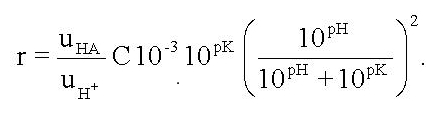# The MIFETM system  for non-invasive measurement of specific fluxes in solution near living plant or animal tissueHome
UTas Biophysics Lab
MIFE user group

MIFE theory
ion flux theory
multivalent ion mobility
ionic mobility values
neutral molecule flux
Methodological isues
H+ flux in buffered media

Hardware
amplifier
microscope
manipulator
System requirements

Software
analysis

Univ of Tas
Eppendorf NP2

## Flux of hydrogen ions in buffered media

The theory is given in detail by Arif et al (1995), Proton flux measurements from tissues in buffered solution.

ABSTRACT: Proton movement across plant cell membranes is part of many important physiological processes. The net proton flux to or from tissues can be determined non-invasively by measuring the proton electrochemical potential gradient in the adjacent solution. In buffered solution, some of the protons crossing the tissue boundary diffuse as protonated buffer whose flux is not included in the flux calculated from the proton (hydrogen ion) electrochemical gradient. In this theoretical paper, it is shown how experimenters can calculate the protonated buffer flux from their measured proton flux in solution. The ratio of these two components of total proton flux depends on the pH of the solution and on the concentration and pK of the buffer. For a given concentration of a buffer which has a single pK, the flux ratio rises with pH when the solution pH is lower than the buffer pK. The slope is about 2 on a log10 scale. As the pH increases above the pK, the flux ratio levels off to approach its maximum. With mixed buffers, or one having two or more pK values, the flux ratios are additive: each buffer acts independently based on its concentration and its pK value. Unbuffered solutions always have buffering effects of water itself and also of carbonates due to carbon dioxide dissolved from the atmosphere. In unbuffered solutions at pH 6, the flux carried by water and carbonate is about 1% the measured proton flux. This validates measurements of proton flux from tissues, made by a number of workers, in unbuffered solutions below pH 6.

Buffers with a single pK.
If there is a flux J of protons from the tissue entering the solution we can express this as the sum of the hydrogen ion flux JH and the protonated buffer flux JHA. The hydrogen ion flux JH is measured by the ion selective microelectrode technique, but JHA is not measured. The sum can be written:
J = JH + JHA. = JH(1 + r),
where r is the ratio  r = JHA/JH.

Using assumptions that are realistic in experimental practice, Arif et al. show that this ratio r may be expressed as their Euqation 13:In this equation, the ratio uHA/uH is the ratio of the mobilities in solution of the protonated buffer and the hydrogen ion. This ratio is also equal to the ratio of their diffusion coefficients D.  The concentration C is the concentration of the buffer as supplied in the solution. It is here expressed in mol m-3, not molar units. pH is the measured pH of the solution and pK is the pK of the buffer. From this equation, the total tissue flux J can be calculated from the measured hydrogen ion flux JH.  This is the calculation that is implemented in the MIFEFLUX software.

Arif et al provide a number of illustrations of this equation.

Mixtures of buffers, and buffers with more than one pK value.
Arif et al  provide an analysis of both of these situations. Neither is implemented in MIFEFLUX.

Maintained by Ian Newman. Date . ©  University of Tasmania.